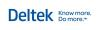You are here: Ajera Help (A-Z) > Year-end > Evaluate your year > About distributing based on costs

# About distributing based on costs

This method allows you to calculate direct personnel expense (DPE) and overhead costs based on direct labor costs from your Expense and Other Income accounts for a more precise calculation than the fixed-percentages method.

Because costs vary over time, Ajera temporarily uses fixed percentages to represent DPE and overhead costs on project reports.

Ajera replaces the fixed-percentage amounts with the cost-based amounts when you distribute DPE/overhead costs.

## DPE

When you distribute DPE based on costs, Ajera performs the following calculations.

 Calculating DPE percent For each project transaction that occurs during the date range specified in the Distribute DPE/Overhead window, it: Divides the cost of the transaction by the total cost of all direct labor transactions in the date range, based on the transaction date. This determines the percentage of the total cost for which the transaction is responsible. Multiplies this percent by the total cost of all general ledger entries in the date range for accounts with project distribution of DPE selected. This determines the DPE cost for the transaction. Divides the DPE cost by the cost of the project transaction. This determines the DPE percent of the transaction.   For example, if the cost of one project transaction is \$60, and the cost of all the transactions is \$220, the transaction is responsible for this percentage of the total cost: \$60 / \$220 = 27.27% If the DPE cost for all general ledger entries is \$75, then the DPE cost for the one transaction is: \$75 x 27.27% = \$20.45           The DPE percent of the \$60 transaction cost is: \$20.45 / \$60 = 34.09% Calculating project cost with DPE The DPE amount added to your project labor cost is calculated as follows: (Cost Amount x DPE Percent) + Cost Amount           For example, if the cost is \$60 and DPE percent is 34.09%, then: (\$60 x 34.09%) + \$60 = \$80.45© 2015 Deltek Inc. All rights reserved.